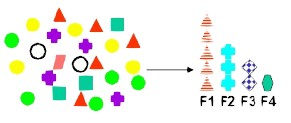# Statistics - Factor Analysis

Factor analysis is a feature extraction statistical method used to describe variability among observed, correlated variables in terms of a potentially lower number of unobserved variables called factors.

For example, it is possible that variations in four observed variables mainly reflect the variations in two unobserved variables.

The information gained about the interdependencies between observed variables can be used later to reduce the set of variables in a dataset.

Factor analysis is related to principal component analysis (PCA), but the two are not identical.

• Latent variable models, including factor analysis, use regression modelling techniques to test hypotheses producing error terms,
• while PCA is a descriptive statistical technique.

There has been significant controversy in the field over the equivalence or otherwise of the two techniques (see exploratory factor analysis versus principal components analysis).

## Model

The observed variables are modelled as linear combinations of the potential factors, plus error terms.

## Documentation / Reference

Discover MoreData Mining - (Feature|Attribute) Extraction Function

Feature extraction is the second class of methods for dimension reduction. dimension reduction It creates new attributes (features) using linear combinations of the (original|existing) attributes. ...